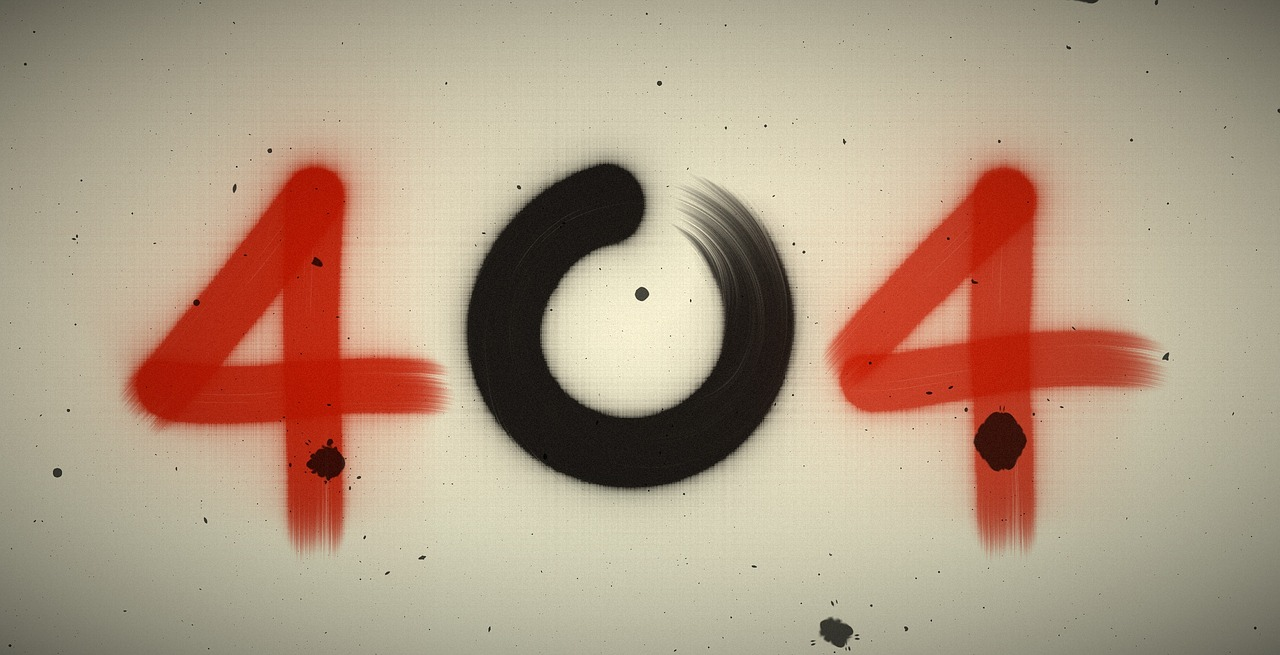## Post per Page

Einstein’s theory of special relativity reflects the unchanging character of the speed of light, among other things. Before Einstein space and time had appeared to be separate and absolute, but after Einstein’s work, space and time became interwoven and relative. The most famous equation of Einstein is mass-energy equation. In this equation Einstein stated that the energy (E) of an object and its mass (m) are not independent perceptions and we can conclude the energy from a known mass by multiplying it twice by the speed of light, or we can conclude the mass from known energy simply by dividing it twice by the speed of light. You can say mass and energy are interchangeable like currency, but one thing we should note here is unlike money, the exchange rate given by two factors of the speed of light is permanent and forever constant.
Image source: Infographic

This exchange factor is so large that a tiny mass goes on tremendously long way in generating energy. In 1905 Einstein stated that the speed of light has been always constant everywhere. There is no difficulty in measuring the speed on earth. As the Einstein’s theory concerns about relative motion so he realized that if the speed of light does not change, then there must be something else that varies. The solution was that the time is not same for all of us. Speed of light is constant but the flow of time is not. The rate of time for the person walking is different from the person standing still. Einstein asked that “can two events occurred simultaneously when they occur at precisely the same time?” The answer  to this question is no they cannot.

Two observers one is standing and another is moving can never agree on a same statement about an event. Suppose there is rail track and an observer with a triangular mirror is standing precisely at equal distance between two poles on the rail track. Imagine that the lightning hit the two poles at once. The observer standing still beside the rail track could see lightning bolt hitting the poles and agrees that the lightning hit the poles at the same time. Now imagine another observer who is in a moving train on the rail track with the same mirror and can see the both poles with a triangular mirror. When second observer, while in moving train, reaches the point, where the first observer was standing, the lightning hits again the two poles and observer on the moving train observers this event. The moving observer does see the lightning bolt hitting the poles simultaneously. He sees the lightning hitting the pole, towards which he is moving, first. Light takes time to move from the pole towards mirror. In that time train travels towards the forward pole and the light from that pole has the shorter distance to cross to reach the mirror. So the two observers one standing still and another is moving cannot agree on “when the light hits the poles”. That’s how Einstein proved that time is relative. Time flows at different rates for each of us. The faster you move the slower your clock will tick as compared to that of a stationary observer.

The effect of this phenomena is hardly observable in our daily life, but if you can move with the 90% of the speed of light the effects will become striking. You would shrink to 44% shorter than your usual length from the point of view of someone watching you outside. At the speed of light length of an object becomes zero and time stands still. So there is no way of crossing this speed limit.

(If you find any error or miscalculation in this article then please feel free to share in comment and if you want to expand this article then comment below)